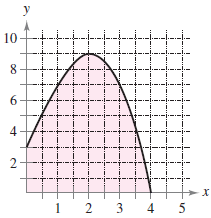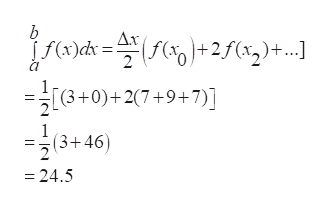y108642234n

Question

Approximate the area of the shaded region using the Trapezoidal Rule and Simpson's Rule with n = 4. (Round your answers to two decimal places.)help_outlineImage Transcriptionclosey 10 8 6 4 2 2 3 4 n fullscreen
Step 1

Given information:

The value of n is 4.

Step 2

Calculation:

From the given interval [0,4] , a=0, b=4 and n=4

delta x=(4-0)/4=1help_outlineImage Transcriptioncloseb Δx f(x)cd = f +2/(x)+...] (3+0)+27+9+7)] (3+46) 24.5 fullscreen
Step 3

For simpson rule

f(0)=3

4f(2)=36

f(4...

Want to see the full answer?

See Solution

Want to see this answer and more?

Our solutions are written by experts, many with advanced degrees, and available 24/7

See Solution
Tagged in

Calculus